# 20212222 2021-2022-2 《Python程序设计》实验二报告

# 20212222 2021-2022-2 《Python程序设计》实验二报告

1.实验内容

2. 实验过程及结果

#设置提示语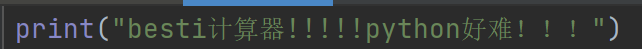#开始循环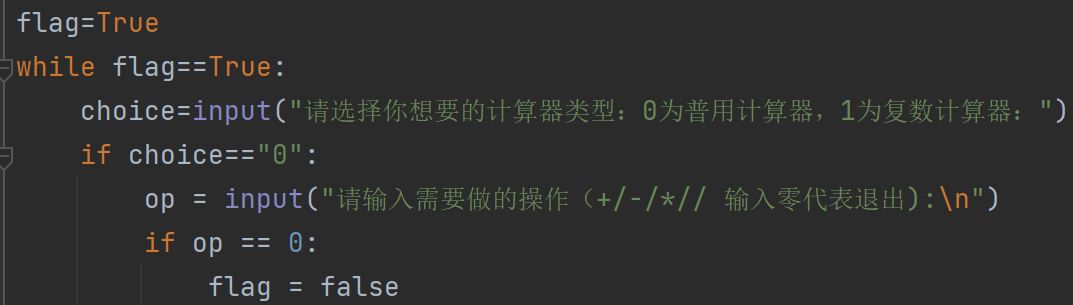#使用判断语句来选择进行的运算操作

1.使用普用还是复数计算器

2.进行什么运算操作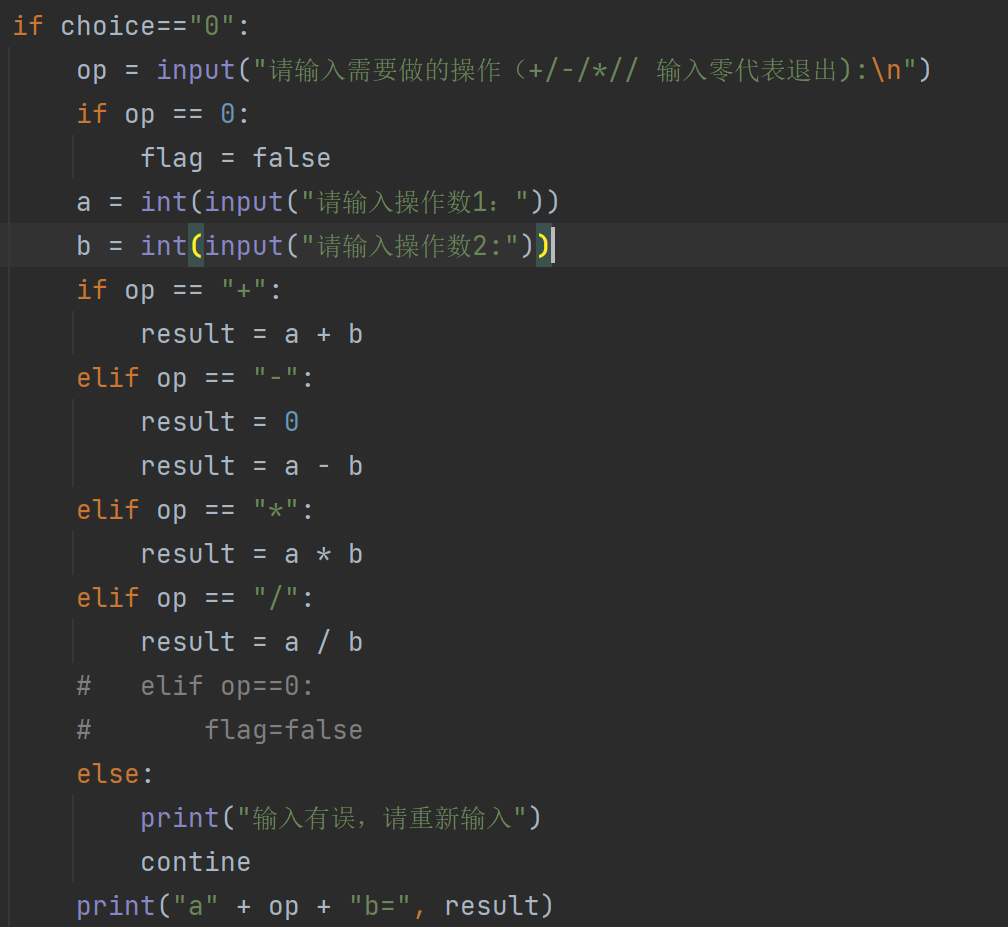#引入复数运算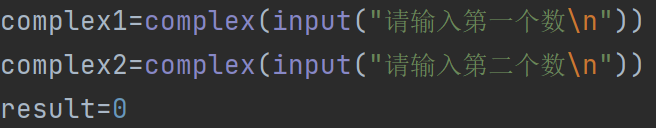#进行运算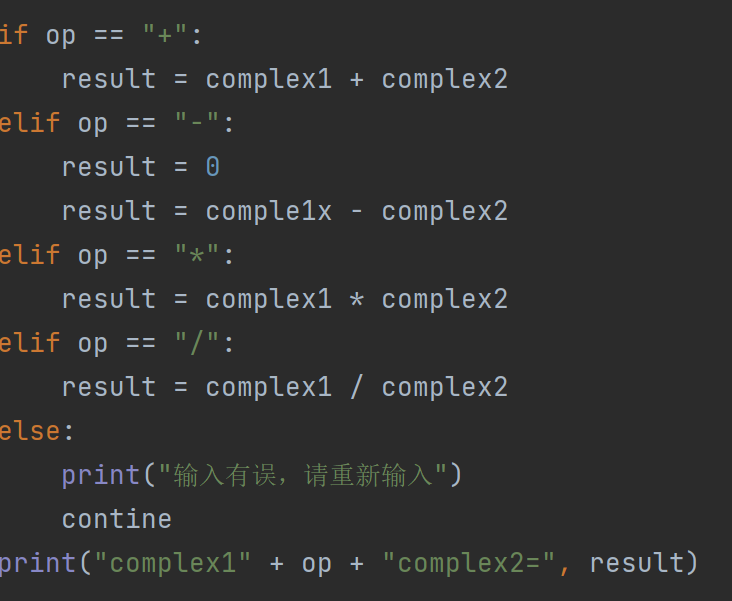3. 实验过程中遇到的问题和解决过程

#flag的位置难以确定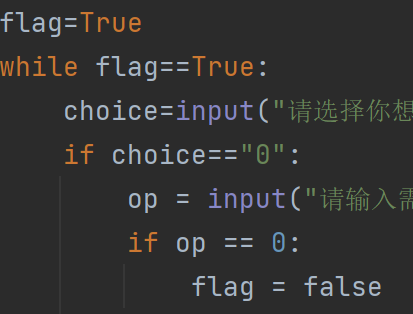#引入输入复数的函数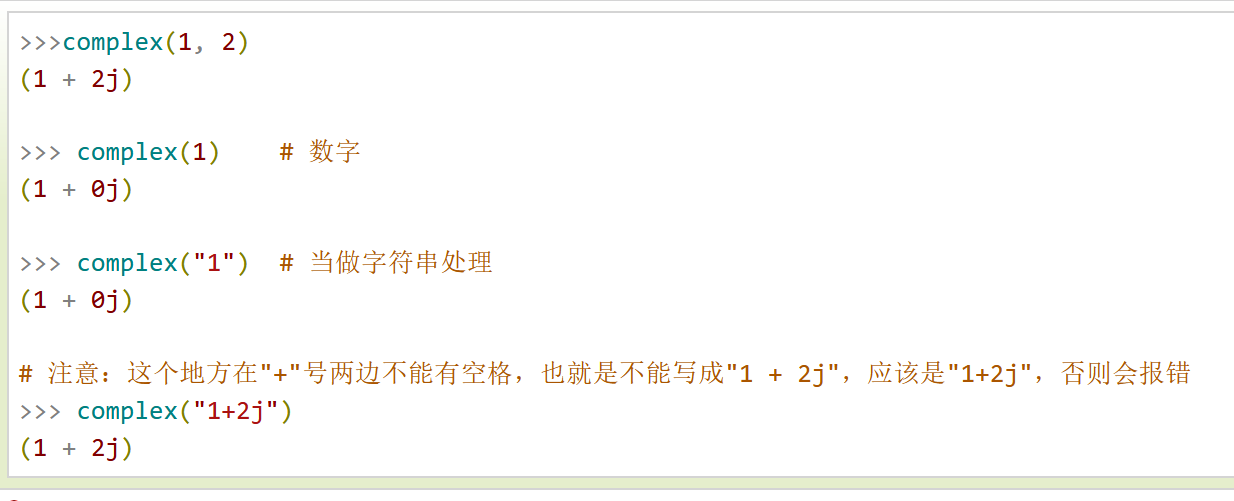#不知道怎么输入复数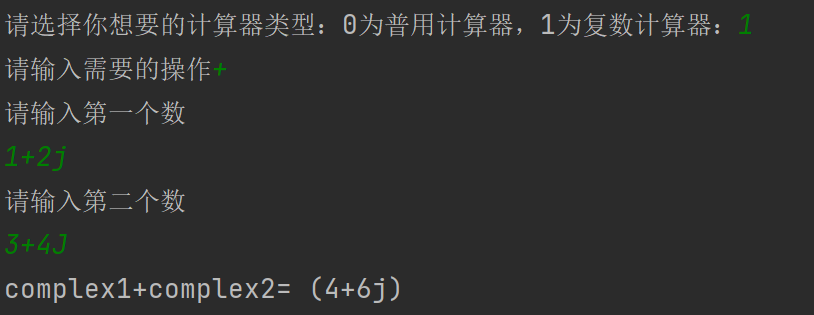#代码

print("besti计算器!!!!!python好难！！！")flag=Truewhile flag==True:    choice=input("请选择你想要的计算器类型：0为普用计算器，1为复数计算器：")    if choice=="0":        op = input("请输入需要做的操作（+/-/*// 输入零代表退出):\n")        if op == 0:            flag = false        a = int(input("请输入操作数1："))        b = int(input("请输入操作数2:"))        if op == "+":            result = a + b        elif op == "-":            result = 0            result = a - b        elif op == "*":            result = a * b        elif op == "/":            result = a / b        #   elif op==0:        #       flag=false        else:            print("输入有误，请重新输入")            contine        print("a" + op + "b=", result)    elif choice=="1":        op=input("请输入需要的操作")        if op=="0":            break        complex1=complex(input("请输入第一个数\n"))        complex2=complex(input("请输入第二个数\n"))        result=0        if op == "+":            result = complex1 + complex2        elif op == "-":            result = 0            result = comple1x - complex2        elif op == "*":            result = complex1 * complex2        elif op == "/":            result = complex1 / complex2        else:            print("输入有误，请重新输入")            contine        print("complex1" + op + "complex2=", result)

附上gitee链接https://gitee.com/hkl200396/tttpython/commit/c67e22dd3f7cb56e0255c7a317403d8068bc30e0

posted @ 2022-04-04 11:56  霸道总柴～  阅读(113)  评论(0编辑  收藏  举报